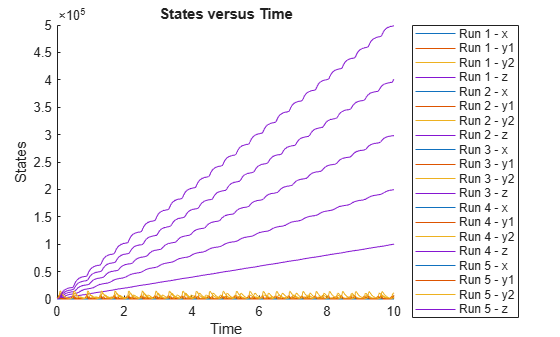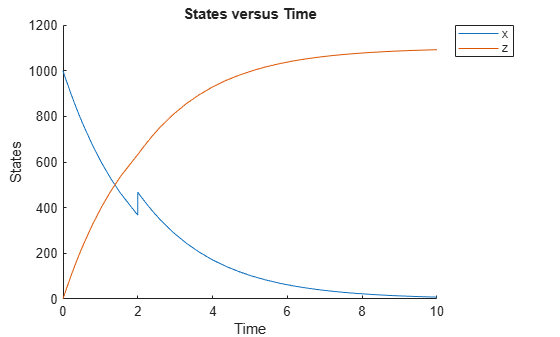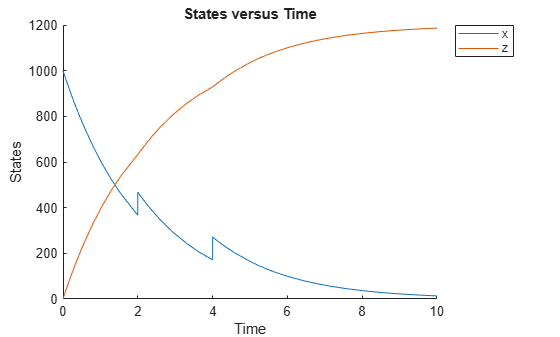# sbioaccelerate

Prepare model object for accelerated simulations

## Syntax

``sbioaccelerate(modelObj)``
``sbioaccelerate(modelObj,csObj)``
``sbioaccelerate(modelObj,dvObj)``
``sbioaccelerate(modelObj,csObj,dvObj)``
``sbioaccelerate(modelObj,csObj,variantObj,doseObj)``

## Description

example

````sbioaccelerate(modelObj)` prepares a model object for an accelerated simulation using its active configuration set (configset), any active variants and active doses. A SimBiology® model can contain multiple configsets with only one being active at any given time. The active configset contains the settings to use in model preparation for acceleration.For accelerated simulations, use `sbioaccelerate` before running `sbiosimulate`. You must use the same model and configset for both functions.Rerun `sbioaccelerate`, before calling `sbiosimulate`, if you modify this model, such as changing reactions or adding events. However, there are exceptions. For details, see When to Rerun Acceleration. NoteIf you are using a `SimFunction object` for simulations, it automatically accelerates the model on its first function evaluation. Hence it is not necessary to run `sbioaccelerate` beforehand. PrerequisitesTo prepare your models for accelerated simulations, install and set up a supported compiler. For details, see Prerequisites for Accelerating Simulations and Analyses. ```

example

````sbioaccelerate(modelObj,csObj)` uses the specified configset object `csObj` and any active variants and active doses. Any other configsets are ignored. If you set `csObj` to empty `[]`, the function uses the active configset.```

example

````sbioaccelerate(modelObj,dvObj)` uses doses or variants specified by `dvObj` and the active configset. `dvObj` can be one of the following: ```Variant object``````ScheduleDose object``````RepeatDose object```array of doses or variants If you set `dvObj` to empty `[]`, the function uses the active configset, active variants, and active doses.If you specify `dvObj` as variants, the function uses the specified variants and active doses. Any other variants are ignored.If you specify `dvObj` as doses, the function uses the specified doses and active variants. Any other doses are ignored.Currently, a particular dose object can only be accelerated with a single model. You cannot use the same dose object for multiple models to be accelerated. You must create a new copy of the dose for each model.```

example

````sbioaccelerate(modelObj,csObj,dvObj)` uses a configset object `csObj` and doses or variants specified by `dvObj`.If you set `csObj` to `[]`, then the function uses the active configset object. If you set `dvObj` to `[]`, then the function uses no variants, but uses active doses.If you set `dvObj` to variants, the function uses the specified variants and active doses. Any other variants are ignored.If you set `dvObj` to doses, the function uses the specified doses and active variants. Any other doses are ignored.```

example

````sbioaccelerate(modelObj,csObj,variantObj,doseObj)` uses a configset object `csObj`, variant object or variant array specified by `variantObj` and dose object or dose array specified by `doseObj`. Any other configset, doses, and variants are ignored. If you set `csObj` to `[]`, then the function uses the active configset object. If you set `variantObj` to `[]`, then the function uses no variants. If you set `doseObj` to `[]`, then the function uses no doses.```

## Examples

collapse all

Load a SimBiology project, named `lotka`, that contains a model `m1`.

`sbioloadproject('lotka','m1')`

Prepare the model for accelerated simulation.

`sbioaccelerate(m1);`

Simulate the model using different initial amounts of species `x`.

```x = sbioselect(m1,'type','species','name','x'); for i=1:5 x.initialAmount = i; sd(i) = sbiosimulate(m1); end```

Plot the results.

`sbioplot(sd);``sbioloadproject radiodecay.sbproj`

Add a new configuration set using a different stop time of 15 seconds.

```csObj = addconfigset(m1,'newStopTimeConfigSet'); csObj.StopTime = 15;```

Prepare the model for accelerated simulation using the new configset object.

`sbioaccelerate(m1,csObj);`

Simulate the model using the same configset object.

```sim = sbiosimulate(m1,csObj); sbioplot(sim);````sbioloadproject radiodecay.sbproj`

Increase the amount of species `x` by 100 molecules at 2 and 4 seconds by adding a schedule dose.

```dObj1 = adddose(m1,'d1','schedule'); dObj1.Amount = 100; dObj1.AmountUnits = 'molecule'; dObj1.TimeUnits = 'second'; dObj1.Time = 2; dObj1.TargetName = 'unnamed.x'; dObj2 = adddose(m1,'d2','schedule'); dObj2.Amount = 100; dObj2.AmountUnits = 'molecule'; dObj2.TimeUnits = 'second'; dObj2.Time = 4; dObj2.TargetName = 'unnamed.x';```

Prepare the model for accelerated simulation using the array of both doses.

`sbioaccelerate(m1,[dObj1,dObj2]);`

Simulate the model using no dose or any subset of the dose array without having to rerun `sbioaccelerate`.

```sim1 = sbiosimulate(m1); sim2 = sbiosimulate(m1,dObj1); sim3 = sbiosimulate(m1,dObj2); sim4 = sbiosimulate(m1,[dObj1,dObj2]);```

Plot the results.

`sbioplot(sim1);``sbioplot(sim2);``sbioplot(sim3);``sbioplot(sim4);``sbioloadproject radiodecay.sbproj`

Get the default configuration set from the model.

`defaultConfigSet = getconfigset(m1,'default');`

Increase the amount of species `x` by 100 molecules at 2 seconds by adding a schedule dose.

```dObj = adddose(m1,'d1','schedule'); dObj.Amount = 100; dObj.AmountUnits = 'molecule'; dObj.TimeUnits = 'second'; dObj.Time = 2; dObj.TargetName = 'unnamed.x';```

Prepare the model for accelerated simulation using the default configset object and added dose object.

`sbioaccelerate(m1,defaultConfigSet,dObj);`

Simulate the model using the same configset and dose objects.

`sim = sbiosimulate(m1,defaultConfigSet,dObj);`

Plot the result.

`sbioplot(sim);``sbioloadproject radiodecay.sbproj`

Add a new configuration set using a different stop time of 15 seconds.

```csObj = m1.addconfigset('newStopTimeConfigSet'); csObj.StopTime = 15;```

Increase the amount of species `x` by 100 molecules at 2 seconds by adding a schedule dose.

```dObj = adddose(m1,'d1','schedule'); dObj.Amount = 100; dObj.AmountUnits = 'molecule'; dObj.TimeUnits = 'second'; dObj.Time = 2; dObj.TargetName = 'unnamed.x';```

Add a variant of species `x` using a different initial amount of 500 molecules.

```vObj = addvariant(m1,'v1'); addcontent(vObj,{'species','x','InitialAmount',500});```

Prepare the model for accelerated simulation using the configset, dose, and variant objects. In this case, the third argument of `sbioaccelerate` must be the variant object.

`sbioaccelerate(m1,csObj,vObj,dObj);`

Simulate the model using the same configset, variant, and dose objects.

`sim = sbiosimulate(m1,csObj,vObj,dObj);`

Plot the result.

`sbioplot(sim);`## Input Arguments

collapse all

SimBiology model, specified as a SimBiology model object. The model minimally needs one reaction or rate rule to be accelerated for simulations.

Configuration set object, specified as a `configset object` that stores simulation-specific information. When you specify `csObj` as`[]`, `sbioaccelerate` uses the currently active configset.

Dose or variant object, specified as one of the following: `ScheduleDose object`, `RepeatDose object`, array of dose objects, `Variant object`, or array of variant objects.

• Use `[]` when you want to explicitly exclude any variant objects from the `sbioaccelerate` function.

• When `dvObj` is a dose object, `sbioaccelerate` uses the specified dose object as well as any active variant objects if available. When you accelerate the model using an array of dose objects, you can simulate the model using any subset of the dose objects from the array.

• When `dvObj` is a variant object, `sbioaccelerate` uses the specified variant object as well as any active dose objects if available. You can use any or no variant input arguments when running `sbioaccelerate`.

Variant object, specified as a `Variant object` or array of variant objects. Use `[]` when you want to explicitly exclude any variant object from `sbioaccelerate`.

Dose object, specified as a `ScheduleDose object`, `RepeatDose object`, or array of dose objects. A dose object defines additions that are made to species amounts or parameter values. Use `[]` when you want to explicitly exclude any dose objects from `sbioaccelerate`.

Note

If you pass in an array of doses to `sbioaccelerate`, you can simulate the model using any subset of doses and do not need to run acceleration again.

### Topics

Introduced in R2010a

## SupportGet trial now